# How to write floor symbol ⌊x⌋ or function like floor() in LaTeX?

Mathematically, the floor function is denoted by the floor ⌊x⌋ symbol and floor(x). The shape of the floor symbol looks like a square bracket ⌊x⌋ without top portions.

Latex does not have any individual commands to mark the floor symbol, you need to use separate commands for both brackets.

Symbol Floor symbol
Type Mathematics
Package Default
Command \lfloor...\rfloor
Example \lfloor x \rfloor → ⌊x⌋

Thus, \lfloor and \rfloor commands define the left and right brackets without top portion.

\documentclass{article}
\begin{document}
$\lfloor x \rfloor$
$\lfloor x^{2} \rfloor$
$\lfloor \frac{1}{x} \rfloor$
\end{document}

Output :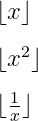Latex does not have any individual pre-defined commands for the floor symbol, but you can create individual commands with that \newcommand.

As a result, you don’t need to write such a large expression for the floor symbol again and again.

\documentclass{article}
\newcommand{\floor}{\lfloor #1 \rfloor}
\begin{document}
$\floor{x}$
$\floor{x^2}$
$\floor{\frac{1}{x}}$
\end{document}

Output :## Big floor symbol in LaTeX

Most of the time mathematical variables are passed as arguments in floor symbols. You need to define the size of the floor symbol according to the size of the mathematical variable.

See the example below, where \lfloor and \rfloor are used for mathematical variables 1/x.

\documentclass{article}
\begin{document}
$\lfloor \frac{1}{x} \rfloor$
$\lfloor \frac{1}{x^2} \rfloor$
$\lfloor \frac{1}{x+1} \rfloor$
\end{document}

Output :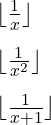However, in this case, the size of 1/x is larger than the floor symbol which is not correct. For this, Big floor symbol or responsive size floor symbol is required.

You need to use the \left and \right commands before the \lfloor and \rfloor commands for a responsive size floor symbol.

\documentclass{article}
\begin{document}
$\left \lfloor \frac{1}{x} \right \rfloor$
$\left \lfloor \frac{1}{x^2} \right \rfloor$
$\left \lfloor \frac{1}{x+1} \right \rfloor$
\end{document}

Output :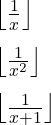If you need to use the big floor symbol more than once, create an independent command with \newcommand. For example

\documentclass{article}
\newcommand{\floor}{\left \lfloor #1 \right \rfloor}
\begin{document}
$\floor{\frac{1}{x}}$
$\floor{\frac{1}{x^2}}$
$\floor{\frac{1}{x+1}}$
\end{document}

Output :You can also use four types of big commands before the \lfloor and \rfloor commands for the big floor symbol.

\documentclass{article}
\begin{document}
$\Bigg \lfloor \bigg \lfloor \Big \lfloor \big \lfloor x \big \rfloor \Big \rfloor \bigg \rfloor \Bigg \rfloor$
\end{document}

Output :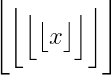Four types of big commands do not work according to the shape of the mathematical variable. For example

\documentclass{article}
\newcommand{\floor}{#2\lfloor #1 #2\rfloor}
\begin{document}
$\floor{x}{\big} \; \floor{x}{\Big} \; \floor{x}{\bigg} \; \floor{x}{\Bigg}$
\end{document}

Output :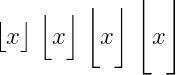So, you saw multiple methods for big floor symbol. But, the best practice is to use \left\lfloor x \right\rfloor syntax.

## Use mathtools package for floor symbol in LaTeX

Instead of denoting the floor symbol with two separate brackets, you can create a new command using the mathtools package and denote it with a single symbol.

\documentclass{article}
\usepackage{mathtools}
\DeclarePairedDelimiter\floor{\lfloor}{\rfloor}
\begin{document}
$\floor{x} \; \floor{\frac{x}{y}} \; \floor{\frac{\floor{x}}{x}}$
$\floor*{x} \; \floor*{\frac{x}{y}} \; \floor*{\frac{\floor*{x}}{x}}$
\end{document}

Output :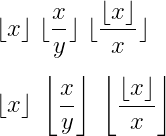If you look at the program above, you will see that \floor and \floor* return two different outputs.

However, using the \floor* command is best practice. Because it defines the size of the floor symbol according to the size of the variable.

Also, you can pass that four types of big commands within \floor command as an optional argument.

\documentclass{article}
\usepackage{mathtools}
\DeclarePairedDelimiter\floor{\lfloor}{\rfloor}
\begin{document}
$\floor[\big]{x} \; \floor[\Big]{x} \; \floor[\bigg]{x} \; \floor[\Bigg]{x}$
\end{document}

Output :## Define floor() function in LaTeX

Most of you will write a mathematical function in a document like text which is not right! For example

\documentclass{article}
\begin{document}
$Floor function : floor(x)$
$Floor function : floor(x)$
\end{document}

Output :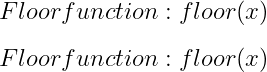Latex uses pre-defined commands or mathrm and mbox commands to write a mathematical function. However, there is no predefined command for the floor function.

\documentclass{article}
\begin{document}
% In math moods use \mathrm{} and \mbox{} commands
$Floor function : \mathrm{floor}(x)$
$Floor function : \mbox{floor}(x)$
\end{document}

Output :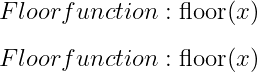## Define floor fraction in LaTeX

When you pass a fractional variable within a floor symbol, the shape of the whole symbol becomes larger. In this case, you must use the \left and \right commands before the \lfloor and \rfloor commands.

As a result, you get a floor symbol of responsive size for fraction variable.

\documentclass{article}
\newcommand{\floor}{\left \lfloor #1 \right \rfloor}
\begin{document}
$\floor{\frac{\floor{x}}{x}}$
$\floor{\frac{x}{y}}$
$\floor{\frac{x}{x+1}}$
$\floor{\frac{x}{\frac{1}{x+1}}}$
\end{document}

Output :Notice the code above, the \frac{num..}{den..} command is used for the fraction part, the numerator and denominator are passed as arguments.

## Use another package like MnSymbol, fdsymbol, and stix

Each package contains the same command to represent the floor symbol. For example

\documentclass{article}
\usepackage{MnSymbol,fdsymbol,stix}
\begin{document}
$\lfloor x \rfloor$
$\left \lfloor \frac{1}{x} \right \rfloor$
\end{document}

Output :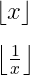So, in my opinion, there is no need to use any package to represent this symbol. Because you can use the same command by default without the help of any package.

## Ceiling symbol in LaTeX

The shape of the ceiling symbol ⌈x⌉  is opposite to the shape of the floor symbol. And \lceil and \rceil commands are used for both brackets. For example

\documentclass{article}
\begin{document}
$\lceil x \rceil$
$\left \lceil \frac{1}{x} \right \rceil$
\end{document}

Output :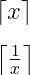So, what you have learned about floor symbols in this tutorial, you can also apply in the case of ceiling symbols. Just you need to use \lceil and \rceil instead of \lfloor and \rfloor.#### Md Jidan Mondal

LaTeX expert with over 10 years of experience in document preparation and typesetting. Specializes in creating professional documents, reports, and presentations using LaTeX.